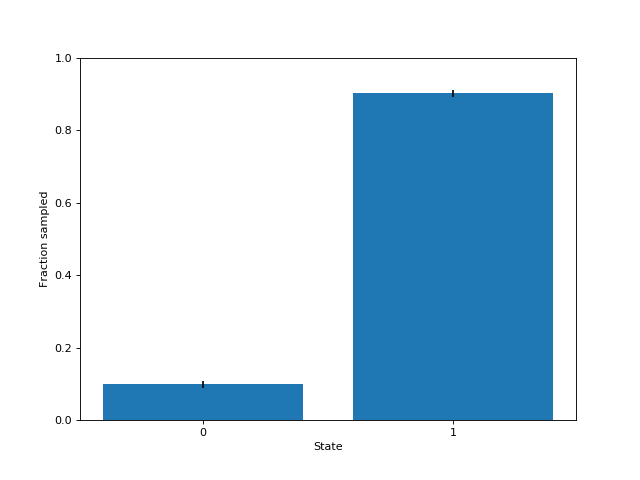# Gumbel max trick

The “Gumbel max trick” is a method for sampling from discrete distributions using only a deterministic function of the distributions’ parameters.

## Introduction

Often in statistics and machine learning, it’s useful to be able to “reparameterize” a problem in a different form. Reparameterization in general refers to any method for changing the representation of parameters in some statistical/probability model, but in this post we’ll focus on one specific type of reparameterization. In particular, we’ll consider reparameterizing distributions so that sampling from them involves a deterministic function of the distribution’s parameters, as well as sampling from a fixed distribution.

As a simple example, we can consider a univariate Gaussian paramterized by a mean $\mu$ and variance $\sigma^2$. Instead of sampling directly from the Gaussian, $x_1 \sim \mathcal{N}(\mu, \sigma^2)$, we could instead take $x_2 = \mu + z \sigma$, where $z \sim \mathcal{N}(0, 1)$. Notice that $x_1$ and $x_2$ will have the same distribution, but the randomness in $x_1$ comes directly from the Gaussian $\mathcal{N}(\mu, \sigma^2)$, so parameters $\mu$ and $\sigma^2$ can’t be dissociated from the randomness. On the other hand, the randomness in $x_2$ comes from a fixed distribution $\mathcal{N}(0, 1)$, and the sample $x_2$ depends on $\mu$ and $\sigma^2$ in a deterministic fashion.

This idea turns out to be useful for a variety of purposes. One of the most popular and recent applications of reparameterization has been in performing variational Bayesian inference using stochastic optimization. Intuitively, reparametrizations such as $x = \mu + z \sigma$ allow us to directly take derivatives of a loss function with respect to model parameters, and so stochastic optimization can be performed.

An important question is: what kinds of distributions can or cannot be reparameterized in this way? The Gaussian case above seems especially nice, but perhaps not generalizable. It turns out that there are certain families of distributions that are amenable to this (e.g., the location-scale family), but many distributions do not admit an easy reparameterization that we know of.

Discrete distributions present a particularly tricky case, and this is the focus of the trick below.

## The Gumbel max trick

How can we sample from an arbitrary discrete distribution using only randomness from a fixed distribution, and a deterministic function of the distribution’s parameters? The “Gumbel max trick” gives the following solution.

Given a discrete distribution over $k$ states with unnormalized probabilities $p_1, p_2, \dots, p_k$, consider the following quantity $x$:

$x = \text{arg}\max \left\{ \log(p_1) + G_1, \log(p_2) + G_2, \dots, \log(p_k) + G_k \right\}$

where $G_i \sim \text{Gumbel}(0, 1)$. Then,

$\mathbb{P}[x = i] = \frac{p_i}{\sum\limits_{j=1}^k p_j}.$

In other words, drawing samples using the above recipe will give us samples from the desired distribution.

Let’s take a closer look at the reparameterization. For each unnormalized state probability $p_i$, we took its logarithm, then added some noise from a Gumbel distribution. Finally, we take the state $i$ that had the maximum value $\log(p_i) + G_i$.

The Gumbel distribution is often used in extreme value theory (i.e., modeling maxima and minima of phenomena). It looks similar to a Gaussian, but has a longer right tail. Intuitively, this means that adding Gumbel noise will randomly bump up lower-probability states some of the time. A more rigorous proof of why this trick works can be found here. Also, note that the Gumbel itself can be reparameterized as $-\log(-\log U)$, where $U \sim \text{Uniform}(0, 1)$.

## Simulations

We can show how this works with a simple simulation. Consider a discrete (Bernoulli) distribution with two states, with unnormalized state-specific probabilities $p_1 = 1$ and $p_2 = 9$. The following code generates samples from this distribution:

n = 1000 # number of samples
num_states = 2

initial_parameterization = [1, 9]
gumbel_rvs = np.random.gumbel(0, 1, size=(n, num_states))
transformed_rvs = np.log(initial_parameterization) + gumbel_rvs
samples = np.argmax(transformed_rvs, axis=1)


Inspecting the samples (here, I ran it multiple times to included error bars), we can see that they approximately follow the desired distribution: state $0$ is chosen 10% of the time, and state $1$ is chosen 90% of the time.• Proof of the Gumbel max trick by Xie Jingyi.
• Kingma, Diederik P., and Max Welling. “Auto-encoding variational bayes.” arXiv preprint arXiv:1312.6114 (2013).
• Maddison, Chris J., Andriy Mnih, and Yee Whye Teh. “The concrete distribution: A continuous relaxation of discrete random variables.” arXiv preprint arXiv:1611.00712 (2016).
• Jang, Eric, Shixiang Gu, and Ben Poole. “Categorical reparameterization with gumbel-softmax.” arXiv preprint arXiv:1611.01144 (2016).